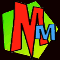# The MODULUS 105 TrickFor this strange trick you need to know what a MODULUS is.

### What is a MODULUS ?

If you divide 14 by 4 you get an answer of 3 plus a remainder of 2.
With some bits of maths, the remainder is the important bit, and if you take a MODULUS, that means you're getting the remainder.
Here's how we'd write down how to get the remainder of dividing 14 by 4: mod4 =2

Here are a few other examples:

mod3 =1     mod7 =2    mod3 =2     mod9 =1     mod26 =1     mod27 =26
And finally mod4 =0 because if you divide 28 by 4 there's no remainder!

Eeek! You don't really want to be dividing by 139, so what you can do is just keep subtracting 139 until you can't go any further. At first you get 435-139= 296 then 296-139 = 157 then 157-139=18. That's it! You can't go any further so mod139 = 18.

### The 105 Trick!

• Ask your friend to think of a mystery number Q between 1 and 105.
• Then ask him to work out [Q]mod3 and tell you the answer. (In other words he has to divide his mystery number by 3 and tell you what the remainder is). Call the answer x
• You then put the answers for x,y and z in this formula: [70x+21y+15z]mod105
• ...and you can work out Q !Example: if the mystery number is 71 then...

71/3 = 23 remainder 2 so mod3=2. This gives x=2.
71/5 = 14 remainder 1 so mod5=1. This gives y=1.
71/7 = 10 remainder 1 so mod7=1. This gives z=1.

Your friend has told you these values for x,y and z (but not the number 71 obviously).
Put these answers in [70x+21y+15z]mod105 to get
[70x2 + 21x1 + 15x1]mod105 = [140 + 21 + 15]mod105 = mod105

Finally to work out mod105 you just do 176-105 =71.